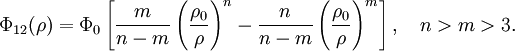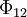# Kihara potential

Jump to: navigation, search

The Kihara potential, developed by Taro Kihara  in 1951, is a non-spherical generalisation of the Lennard-Jones model. It is given by$\Phi_{12}(\rho)=\Phi_0 \left[\frac{m}{n-m}\left(\frac{\rho_{0}}{\rho}\right)^n-\frac{n}{n-m}\left(\frac{\rho_{0}}{\rho}\right)^m\right], ~~~ n>m>3.$

where$\Phi_{12}$ is the intermolecular pair potential, and$\rho$ is the shortest distance between molecule cores.

.